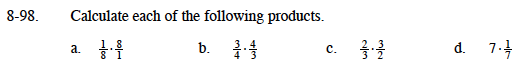### Home > MC1 > Chapter 8 > Lesson 8.3.2 > Problem8-98

8-98.Multiply the numerators by one another and the denominators by one another.

$\left(\frac{1}{8}\right)\left(\frac{8}{1}\right)=\frac{(1)(8)}{(8)(1)}$

Simplify the fraction.

$\frac{8}{8}=1$

1

Follow the same steps as in part (a).

1

Parts (c) and (d) are very similar to part (a).